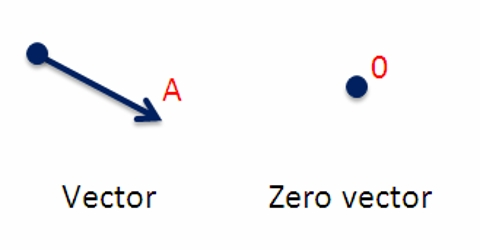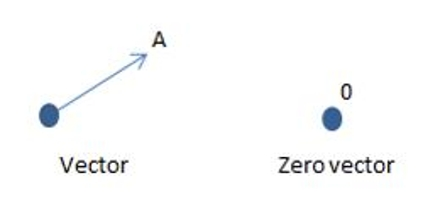Physics

# Null or Zero VectorSuppose, two persons at the ends of a rope are pulling with the same force. Then the resultant of that tension will be a null vector. In other words, the vector whose magnitude is zero is called a null or zero vector.The initial and the end point of a zero vector is the same. It is denoted by Ō or O. By adding a vector with its opposite vector or by subtracting two equal vectors, a null vector is obtained. It has no particular direction. Generally, a null vector is either equal to resultant of two equal vectors acting in opposite directions or multiple vectors in different directions.

Graphically, zero vector or null vector represent just a point whose starting point and terminating point is same.